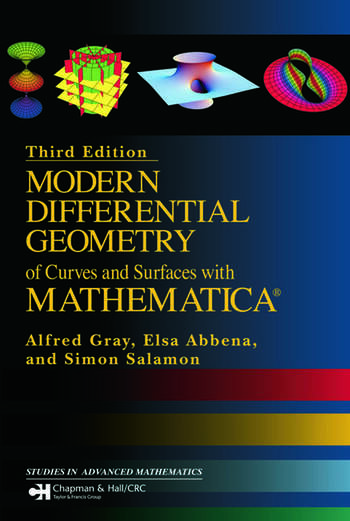# Modern Differential Geometry of Curves and Surfaces with Mathematica

## 3rd Edition

Elsa Abbena, Simon Salamon, Alfred Gray

Chapman and Hall/CRC
Published June 21, 2006
Textbook - 1016 Pages - 531 B/W Illustrations
ISBN 9781584884484 - CAT# C4487
Series: Textbooks in Mathematics

For Instructors Request Inspection Copy

USD\$135.00

FREE Standard Shipping!

## Preview

### Summary

Presenting theory while using Mathematica in a complementary way, Modern Differential Geometry of Curves and Surfaces with Mathematica, the third edition of Alfred Gray’s famous textbook, covers how to define and compute standard geometric functions using Mathematica for constructing new curves and surfaces from existing ones. Since Gray’s death, authors Abbena and Salamon have stepped in to bring the book up to date. While maintaining Gray's intuitive approach, they reorganized the material to provide a clearer division between the text and the Mathematica code and added a Mathematica notebook as an appendix to each chapter. They also address important new topics, such as quaternions.

The approach of this book is at times more computational than is usual for a book on the subject. For example, Brioshi’s formula for the Gaussian curvature in terms of the first fundamental form can be too complicated for use in hand calculations, but Mathematica handles it easily, either through computations or through graphing curvature. Another part of Mathematica that can be used effectively in differential geometry is its special function library, where nonstandard spaces of constant curvature can be defined in terms of elliptic functions and then plotted.

Using the techniques described in this book, readers will understand concepts geometrically, plotting curves and surfaces on a monitor and then printing them. Containing more than 300 illustrations, the book demonstrates how to use Mathematica to plot many interesting curves and surfaces. Including as many topics of the classical differential geometry and surfaces as possible, it highlights important theorems with many examples. It includes 300 miniprograms for computing and plotting various geometric objects, alleviating the drudgery of computing things such as the curvature and torsion of a curve in space.

#### Instructors

We provide complimentary e-inspection copies of primary textbooks to instructors considering our books for course adoption.